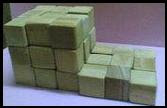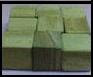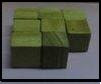## Sunday, April 27, 2008

Activity(3)
Aim: Using the unit cubes verify the algebraic identity
(a-b)^3 = a^3 -3a^2b+3ab^2- b^3.
Material required: Unit cubes
Procedure:
1) Let a=3 and b=1.
(a-b)^3 = (2) ^3
To represent (a-b) ^3 make a cube of dimension (a-b) x (a-b) x (a-b) i.e. 2x2x2 cubic units

To represent (a) ^3 make a cube of dimension 3x3x3 cubic units3) To represent 3ab^2 make 3 cuboids of dimension 3x1x1 cubic units.4) To represent a^3 + 3ab^2, join the cube and the cuboids formed in steps 2 and 3.5) To represent a^3 + 3ab^2- 3a^2b extract from the shape formed in the previous step 3 cuboids of dimension 3x3x1.6) To represent a^3 + 3ab^2- 3a^2b-b^3 extract from the shape formed in the previous step 1 cube of dimension 1x1x1.7) Arrange the unit cubes left to make a cube of dimension2x2x2 cubic units.Observation:
The number of unit cubes in a^3 = …27…..
The number of unit cubes in 3ab^2 =…9……
The number of unit cubes in 3a^2b=…27…
The number of unit cubes in b^3 =…1…
The number of unit cubes in a^3 - 3a^2b + 3ab^2- b^3 =..8..
The number of unit cubes in (a-b) ^3 =…8...
The number of unit cubes in (a-b) ^3 = number of unit cubes

in a^3 -3a^2b+3ab^2-b^3.

Result:
(a-b)^3 = a^3 -3a^2b+3ab^2- b^3.